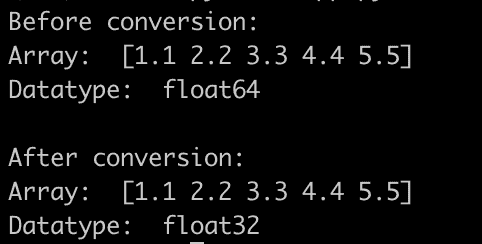# Tensorflow ValueError Failed to convert a NumPy array to a Tensor Unsupported object type float

Tensorflow ValueError Failed to convert a NumPy array to a Tensor Unsupported object type float error occurs when you are “converting a NumPy array into a TensorFlow tensor because it contains an unsupported data type (in this case, float).”

Here are the solutions that you can work on to fix the ValueError Failed to convert a NumPy array to a Tensor Unsupported object type float error.

1. Convert the Data Types
2. Reshaping the data
3. Using “tf.constant” or “tf.convert_to_tensor”
4. Check for “NaN or Inf” values

## Solution 1: Convert the data types

The essential step is to double-check the data types of your NumPy arrays and the Tensorflow functions you’re using. Ensure that your arrays’ data types match the data types expected by the functions.

TensorFlow prefers float32 for its operations. If your array is in a different float type, such as float64, you might need to convert it. You can do this with the “numpy.astype()” method:

``array = array.astype('float32')``

See the below code for more understanding:

``````import numpy as np

# Let's create a numpy array of float64 type
array = np.array([1.1, 2.2, 3.3, 4.4, 5.5], dtype='float64')

# Check the current datatype
print("Before conversion:")
print("Array: ", array)
print("Datatype: ", array.dtype)

# Convert the array to float32 type
array = array.astype('float32')

# Check the datatype after conversion
print("\nAfter conversion:")
print("Array: ", array)
print("Datatype: ", array.dtype)
``````

Output## Solution 2: Reshaping the Data

TensorFlow often expects arrays of a certain shape, mainly when used to create tensors for machine learning models. Ensure that your array has the correct number of dimensions and that each dimension is of the correct size.

You can check the shape of a numpy array using the .shape attribute:

``````import numpy as np

array = np.array([[1, 2, 3], [4, 5, 6]])
print(array.shape)

reshaped_array = array.reshape((6,))
print(reshaped_array.shape)``````

Output

``````(2, 3)
(6,)
``````

This reshapes the 2D array into a 1D array with 6 elements. Please keep in mind that the total number of elements must remain the same after the reshaping.

## Solution 3: Using “tf.constant” or “tf.convert_to_tensor”

Both tf.constant and tf.convert_to_tensor are used to create TensorFlow tensors from data (like arrays). They work in similar ways and can often be used interchangeably. Here’s how you can use them:

### Using tf.constant

``````import tensorflow as tf
import numpy as np

# Create a numpy array
array = np.array([1, 2, 3, 4, 5], dtype='float32')

# Convert the array to a tensor using tf.constant
tensor = tf.constant(array)

print(tensor)
``````

Output

``tf.Tensor([1. 2. 3. 4. 5.], shape=(5,), dtype=float32)``

### Using tf.convert_to_tensor

``````import tensorflow as tf
import numpy as np

# Create a numpy array
array = np.array([1, 2, 3, 4, 5], dtype='float32')

# Convert the array to a tensor using tf.convert_to_tensor
tensor = tf.convert_to_tensor(array)

print(tensor)
``````

Output

``tf.Tensor([1. 2. 3. 4. 5.], shape=(5,), dtype=float32)``

## Solution 4: Check for NaN or Inf values

TensorFlow might have trouble converting arrays that contain NaN (Not a Number) or Inf (Infinity) values. You can check for these using the “numpy.isnan()” and “numpy.isinf()” functions and replace them if necessary.

``````import numpy as np

# Let's create a numpy array with some NaN and Inf values
array = np.array([1, 2, np.nan, 4, np.inf, 6], dtype='float32')

# Check if there are any NaN or Inf values in the array
has_nan = np.isnan(array).any()
has_inf = np.isinf(array).any()

print("Contains NaN values:", has_nan)
print("Contains Inf values:", has_inf)
``````

Output

``````Contains NaN values: True
Contains Inf values: True``````

## Related posts

Cannot convert a symbolic Tensor to a Numpy Array

RuntimeError: Can’t call numpy() on Variable that requires grad. Use var.detach().numpy() instead

AttributeError: module ‘numpy’ has no attribute ‘long’

AttributeError: module ‘numpy’ has no attribute ‘int’

AttributeError: ‘Tensor’ object has no attribute ‘numpy’

This site uses Akismet to reduce spam. Learn how your comment data is processed.